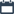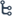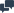## Thursday, February 27, 2020

•February 27, 2020
••, , ,
•No comments

## The LCM of two numbers is 432 and their HCF is 36. If one of the numbers is 108, then find the other number

The LCM of two numbers is 432 and their HCF is 36. If one of the numbers is 108, then find the other number
The other number                  = 15552 ÷ 108
=   144
Answer : the other number is 144

## Categories